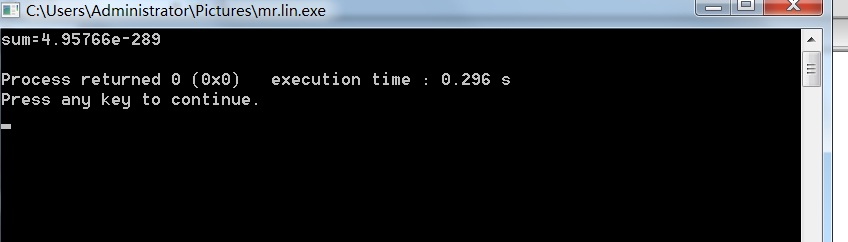• SELECT max(pp.totalPrice) FROM ( SELECT sum(oi.product_price) totalPrice FROM order.item oi GROUP BY oi.id ) as pp SELECT oi.id, oi. sum(oi.product_price) AS pp ... order.i
SELECT
max(pp.totalPrice)
FROM
(
SELECT
sum(oi.product_price) totalPrice
FROM
order.item oi
GROUP BY
oi.id
) as ppSELECT
oi.id,
oi.
sum(oi.product_price) AS pp
FROM
order.item oi
GROUP BY
oi.id
order by pp desc limit 1

展开全文mysql
• 39. 组合总和 class Solution { int len=0; public List<List<Integer>> combinationSum(int[] candidates, int target) { List<List<Integer>> ans=new ArrayList<>(); //DFS...
39. 组合总和

class Solution {
int len=0;
public List<List<Integer>> combinationSum(int[] candidates, int target) {
List<List<Integer>> ans=new ArrayList<>();
//DFS模拟一个栈
len=candidates.length;
// 先将数组排序，这一步很关键
// 排序是为了提前终止搜索
Arrays.sort(candidates);
if(candidates==null||len==0) return ans;
dfs(candidates,target,0,path,ans);
return ans;
}
private void dfs(int[] candidates,int target,int begin,LinkedList<Integer> path,List<List<Integer>> ans){
//结束条件。
if(target==0){
return;
}
for(int i=begin;i<len;i++){
if(candidates[i]>target) break;

// 可以重复，传递下去的是 i
dfs(candidates,target-candidates[i],i,path,ans);
path.removeFirst();
}

}
}

40. 组合总和 II

class Solution {
int len=0;
public List<List<Integer>> combinationSum2(int[] candidates, int target) {
List<List<Integer>> ans=new ArrayList<>();
//DFS模拟一个栈
len=candidates.length;
// 先将数组排序，这一步很关键
// 排序是为了提前终止搜索
Arrays.sort(candidates);
if(candidates==null||len==0) return ans;
dfs(candidates,target,0,path,ans);
return ans;
}
private void dfs(int[] candidates,int target,int begin,LinkedList<Integer> path,List<List<Integer>> ans){
//结束条件。
if(target==0){
return;
}
for(int i=begin;i<len;i++){
if(candidates[i]>target) break;
//【修改1】 小剪枝
if (i > begin && candidates[i] == candidates[i - 1]) {
continue;
}

//【修改2】  不可以重复，传递下去的是 i+1
dfs(candidates,target-candidates[i],i+1,path,ans);
path.removeFirst();
}

}
}


展开全文• ## 求阶乘总和

千次阅读 2014-10-28 08:35:31
代码： /* ... *All rights reserved. *文件名称：sum.cpp *作 者：林海云 ...*完成日期：2014年10月28日 ...*问题描述： 1！+2！+3!+......20！ *程序输出：总和 */ #include using namespace st
                   代码：
/*
*文件名称：sum.cpp
*作    者：林海云
*完成日期：2014年10月28日
*版 本 号：v1.0
*
*问题描述： 求1！+2！+3!+......20！
*程序输出：总和
*/
#include <iostream>
using namespace std;
int main()
{
double i,f=1,sum=0;
while(i<=20)
{
f=f*i;
sum=sum+f;
i++;
}
cout<<"sum="<<sum<<endl;
return 0;
}


运行结果：总结：按照公式求和，就运行出这么个结果，醉了！数不是一般的大。

展开全文c++ namespace
• jquery table 横竖列的求总和，jquery table 横竖列的求总和，jquery table 横竖列的求总和 参考 var lastTr = []; $("#table").find("tr").each(function(j, e){ if(j == 0){ return true • 求总和 Problem : 176 Time Limit : 1000ms Memory Limit : 65536K description 给出一列数(s1,s2,s3...sn)，从1到m所有数但不包括给出的数(s1到sn)的总和。例：n=4，s1到s4分别是2,4,7,9，m=5。总和... 求总和 Problem : 176 Time Limit : 1000ms Memory Limit : 65536K description 给出一列数(s1,s2,s3...sn)，求从1到m所有数但不包括给出的数(s1到sn)的总和。例：n=4，s1到s4分别是2,4,7,9，m=5。总和是1+3+5=9。 n,m都是小于1000的正整数。给出数从小到大排列，且不会重复，s1到sn可能会大于m,但都小于100000。 input 多组测试数据，第一行是一个数n，第二行n个数，s1到sn。第三行是m。 output 输出一个数，1到m的但不包括给出数的总和。 sample_input 4 2 4 7 9 5 5 2 4 6 9 10 10 sample_output 9 24 hint source 分析： 做标记。。 代码： //到家第一发 2016 - 1 - 17 #include <iostream> using namespace std; int main() { int sum,n,m,a,blog; while(cin>>n) { for(int i=1;i<=n;i++) cin>>a[i]; cin>>m; sum=0; for(int i=1;i<=m;i++) { blog=0; for(int j=1;j<=n;j++) if(i==a[j]) { blog=1; break; } if(blog==0) sum=sum+i; } cout<<sum<<endl; } return 0; } ` 展开全文• LeetCode113 路径总和 给定一个二叉树和一个目标和，找到所有从根节点到叶子节点路径总和等于给定目标和的路径。 说明:叶子节点是指没有子节点的节点。 5 / \ 4 8 / / \ 11 13 4 / \ /... • 先通过group by得到每个分类别的总和，再通过开窗函数计算整体的总和，两者相除就得到占比了。 当over()后不加任何内容时，就是对所有的数据进行汇总。 1.使用over (partition by)得到总计 select sites_id,channel_...数据分析 大数据 • Excel固定总和的组合.rar,本例内容包括：1、根据指定的总和数，产生不重复随机数；2、根据指定的总和数，找出数据组合。Excel应用 • 求总和 例子 每一本图书的销售总额 例子 年度图书销售总额 每一本图书在某年的销售总额 这里用到了链式调用 查询集具有annotate方法 • forEach 无法用break,可以用try/catch中thrownew...// forearch求总和 var numbers = [1,2,3,4,5,6]; var num = 0; // 方式一 // numbers.forEach(function(i){ // num += i; // }) // document.write(num)//21 // ... • 数据结构-二叉树-路径总和 问题描述 给定一个二叉树和一个目标和，判断该树中是否存在根节点到叶子节点的路径，这条路径上所有节点值相加等于目标和。 说明: 叶子节点是指没有子节点的节点。 示例 给定如下二叉树...数据结构 • 1-100所有数相加的总和 1-100所有偶数相加的总和 1-100所有奇数相加的总和 */ public static void main(String [] args){ int i; int sum1=0,sum2=0,sum3=0; for(i=0;i<=100;i++){ sum1+=i;//计算1-100java • ## MapReduce之求各个部门工资的总和 千次阅读 多人点赞 2021-05-17 22:05:06 MapReduce之各个部门工资的总和一、需求说明二、测试数据三、实现步骤四、打包上传到集群中运行 一、需求说明 利用MapReduce程序出各个部门工资的总和 二、测试数据 员工信息表：下载地址 表字段说明： ...hadoop • ## 求列表内元素总和 千次阅读 2019-10-26 11:19:32 total = 0 list1 = [2, 3, 5] for x in range(0, len(list1)): total = total + list1[x] print( total) • 1.代码 package day07; public class ArrayExer1 { public static void main(String[] args) { //数组练习: int[][] arr=new int[][]{{3,5,8},{12,9}...//记录总和 for (int i = 0; i < arr.length; i++) { fo • C++一个整数的各位数字总和算法C++一个整数的各位数字总和算法完整源码（定义，实现，main函数测试） C++一个整数的各位数字总和算法完整源码（定义，实现，main函数测试） int sum_of_digits(int num) { // ... • 如果我们列出10以下的所有...出1000以下3或5的所有倍数的总和。 public static void main(String[] args) { int sum=0; for(int i=0;i&lt;1000;i++){ if(i%3==0||i%5==0){ System.out.pri... • //某字段的总和$res2=mysql_query("select sum(price) as sumyy from services whereDATE_FORMAT(laifangtime, '%Y-%m-%d') >= '$starttime' andDATE_FORMAT(laifangtime, '%Y-%m-%d')$endtime' ".$r1."".$r2...大数据 mapreduce java hadoop
• 有一张表tbinfo,关键字段部门dname,发布时间ctime 数据类似如下：   ; height: 82px;" border="0"> <td>dname ...<p>select to_char(ctime,'yyyymm') ym,dname,count(*) infocount from tbinfo group by to_char...oracle
• 假如现在有一张表（表a）简化如下 [img=... 现在我要求编号相同的数量总和 就是显示以下的效果 [img=https://img-bbs.csdn.net/upload/201408/12/1407813194_968927.jpg][/img] 请问sql语句肿么写
• #include void sum(int begin,int end) //定义一个没有返回值的函数名sum,并定义两个为整形的形参begin和end. { int i; //i为循环次数 int sum = 0; for (i=begin;i;i++) //i等于开始数,如果i小于等于结束数,...
• public class 取得数组总和 { public static void main(String[] args) { int[] data= {12,43,8,9,1,46,32,23,65,78,1,1}; double s=0; for(int x=0;x<data.length;x++) { s=s+da...
• <!DOCTYPE html> <html lang="en"> <head> <meta charset="UTF-8"> <meta name="viewport" content="width=device-width, initial-scale=1.0"> <...script>
• 快速求总和 最大值 最小值 和 平均值 NSArray *array = [NSArray arrayWithObjects:@"2.0", @"2.3", @"3.0", @"4.0", @"10", nil]; CGFloat sum = [[array valueForKeyPath:@"@sum.floatValue"] float...
• 出数组中元素的总和Description: 描述： Given an array of n elements. Find three elements such that their sum is equal to given element K. 给定n个元素的数组。 找到三个元素，使其总和等于给定元素K。 ...算法 c++ python 数据结构 leetcode
• 读取文件，提取数字，求总和 文件内容格式 第 0 组个数：9, Time: 0.037400 第 1 组个数：585, Time: 0.430600 第 2 组个数：27, Time: 0.023400 目标 0.037400 + 0.430600 + 0.023400 = ? 代码 #...string fp
• * 需求：1-100中7的倍数的总和 */ int a = 1; int b = a * 7; int c = 0; while(a ){ c = c + b; a++; } System.out.println(c); } 我想知道我设置3个变量计算的结果...java
• public int getMobileCount(Date createtime){ StringBuilder sql= new StringBuilder(); sql.append("SELECT COUNT(APPLY.ID) FROM A "); sql.append("INNER JOIN B ON (A.INTEGRATEACTIVITY = B.ID)");...select sql...